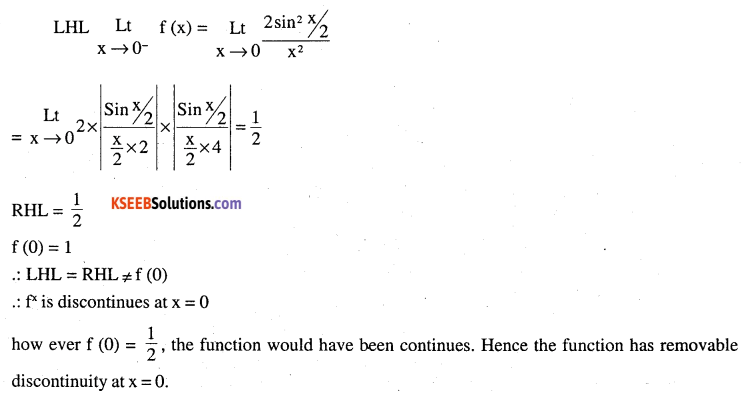# 2nd PUC Maths Question Bank Chapter 6 Application of Derivatives Miscellaneous Exercise

Students can Download Maths Chapter 6 Application of Derivatives Miscellaneous Exercise Questions and Answers, Notes Pdf, 2nd PUC Maths Question Bank with Answers helps you to revise the complete Karnataka State Board Syllabus and score more marks in your examinations.

## Karnataka 2nd PUC Maths Question Bank Chapter 6 Application of Derivatives Miscellaneous Exercise

Question 1.
Using differentials, find the approximate value of each of the following

(a) $$\left(\frac{17}{81}\right)^{1 / 4}$$(b) (33)-1/5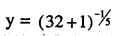Question 2.
Show that the function given by log has maximum at $$f(x)=\frac{\log x}{x}$$ has maximum at x = e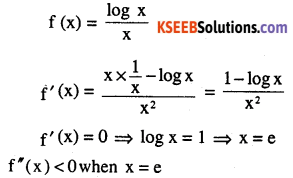Question 3.
The two equal sides of an isosceles triangle with fixed base b are decreasing at the rate of 3 cm per second. How fast is the area decreasing when the two equal sides are equal to the base ?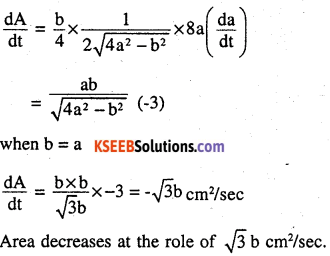Question 4.
Find the equation of the normal to curve y2 = 4x which passes through the point (1, 2).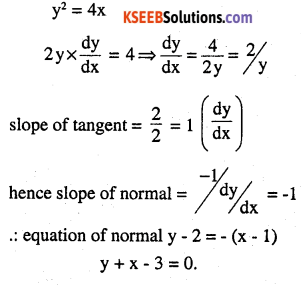Question 5.
Show that the normal at any point 6, to the curve x = a cos θ +a θ sin θ ,y = a sin θ –
a θ cos θ is at a constant distance from the origin.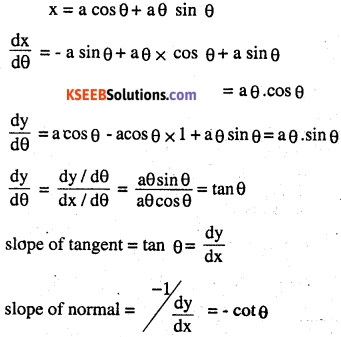∴ equation of normal is
y – (a sin θ – a θ cos θ)
= – cot θ (x – a cos θ – a θ sin θ)
y + x cot θ= a cot θ cos θ+a θ cot θ sin θ – a θ cos θ
$$y+\frac{x \cos \theta}{\sin \theta}=\frac{a \cos ^{2} \theta}{\sin \theta}+\frac{a \sin ^{2} \theta}{\sin \theta}$$
x cos θ a cos2θ, a sin2θ
normal form is x cos θ+ y sin θ = p when p is the normal from the normal to the given curve is at a consistent distance ‘a’ from the origin.Question 6.
Find the intervals in which the function f given by
$$f(x)=\frac{4 \sin x-2 x-x \cos x}{2+\cos x}$$
(i) increasing
(ii) decreasing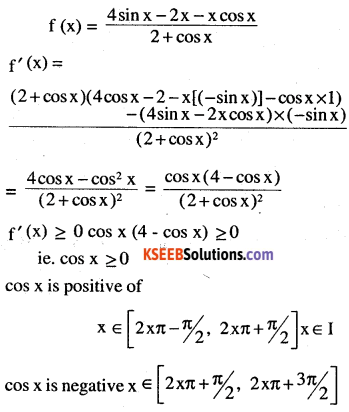Question 7.
Find the intervals in which the function f given by
x3 + -1/x3, x ≠ 0
(i) increasing
(ii) decreasing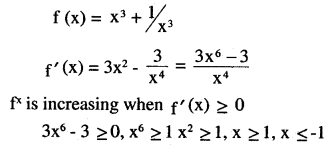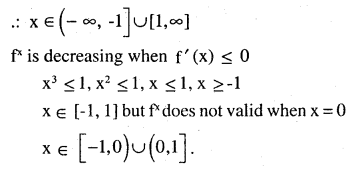Question 8.
Find the maximum area of an isosceles triangle inscribed in the ellipse
$$\frac{x^{2}}{a^{2}}+\frac{y^{2}}{b^{2}}=1$$ with its vertex at one end of the major axis.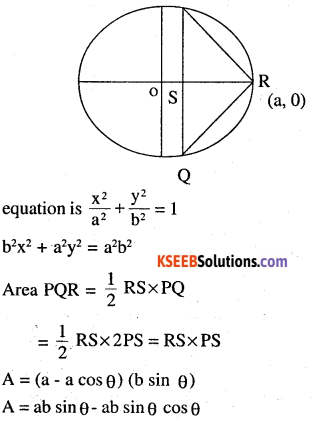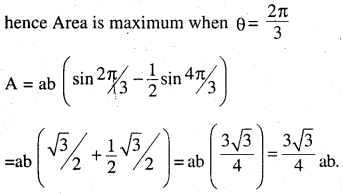Question 9.
‘A tank with rectangular base and rectangular sides, open at the top is to be constructed so that its depth is 2 m and volume is 8 m3. If building of tank costs ₹ 70 per sq metres for the base and ₹ 45 per square metre for sides. What is the cost of least expensive tank?Question 10.
The sum of the perimeter of a circle and square is k, where k is some constant. Prove that the sum of their areas is least when the side of square is double the radius of the circle.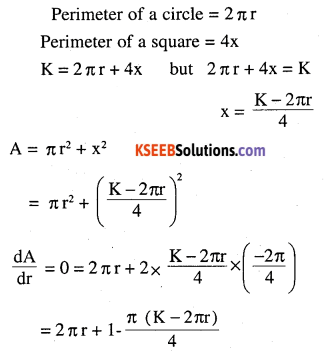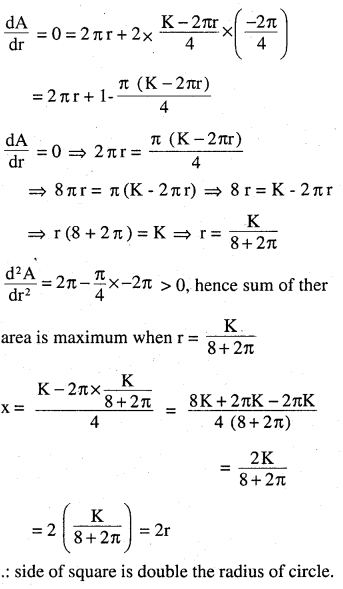Question 11.
A window is in the form of a rectangle surmounted by a semicircular opening. The total perimeter of the window is 10 m. Find the dimensions of the window to admit maximum light through the whole opening.Question 12.
A point on the hypotenuse of a triangle is at distance a and b from the sides of the triangle. Show that the maximum length of the hypotenuse is
$$\left(a^{\frac{3}{2}}+b^{\frac{2}{3}}\right)^{\frac{3}{2}}$$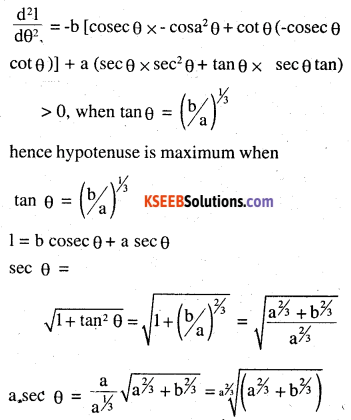Question 13.
Find the points at which the function f given by f (x) = (x – 2)4 (x + 1)3 has
(i) local maxima
(ii) local minima
(iii) point of inflexion
f (x) = (x – 2)4 (x + 1)3
f’ (x) = (x – 2)4 3 (x + 1)2 + (x + 1)3 4(x – 2)3
= (x – 2)3 (x + 1) [3 (x -2) + 4 (x + 1)]
= (x – 2)3 (x + 1)2 [3x – 6 + 4x + 4]
= (x – 2)3 (x + 1)2 [7x – 2]
f’ (x) = 0 ⇒ x = 2, x = -1, x = 2/7
f’ (x)=(x – 2)3 (x+1)2 x 7 + (x – 2)3 (7x – 2) 2 (x +1) + (x + 1)2 (7x – 2)3 (x – 2)2
here second demivalive test fails as f” (x) = 0 then we apply first demivalive test
At x = 2 f'(x) changes from negative to positive.
∴ function has minimum value and the local minimum value is f (2) = 0
At x = -1
f’ (x) does not change (positive to negative)
∴ fx has neither maximum value nor minimum value.
∴ x = -1 is the point of inflection at x = 2/7
f’ (x) change from positive to negative
hence function has been maximum at x = 2/7 and local maximum value is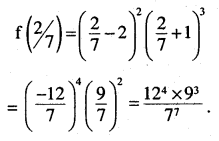Question 14.
Find the absolute maximum and minimum values of the function f given by
f (x) = cos2 x + sin x, x ∈ [o,π]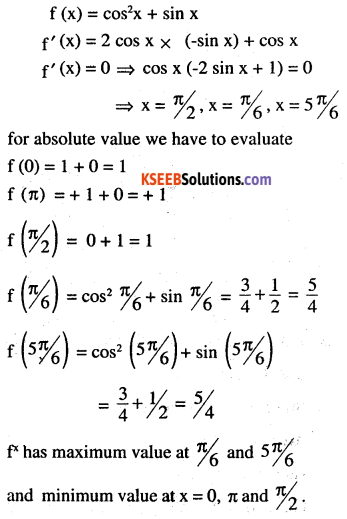Question 15.
Show that the altitude of the right circular cone of maximum volume that can be inscribed in a sphere of radius r is $$\frac{4 r}{3}$$
Let the radius of sphere be ‘r’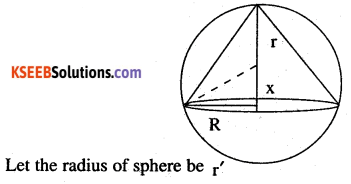Question 16.
Let f be a function defined on [a, b] such that f’ (x) > 0, for all xe (a, b). Then prove that f is an increasing function on (a, b).
Let x1, x2 be any two real number [a, b] such that x1 <  x2, then f (x) satisfies the conditions of L.M.V theorem. ∋ C ∈  (a, b)Question 17.
Show that the height of the cylinder of maximum volume that can be inscribed in sphere of radius R is $$\frac{2 \mathbf{R}}{\sqrt{3}}$$. Also find the maximum volume.
Let r be the radius of the cylinder and height 2xQuestion 18.
Show that height of the cylinder of greatest volume which can be inscribed in a right circular cone of height h and semi vertical angle a is one-third that of the cone and α the greatest volume of cylinder is $$\frac{4}{27} \pi \mathrm{h}^{3}$$
Height of cone – h
Height of cylinder – yChoose the correct answer in the Exercises from 19 to 24.

Question 19.
A cylindrical tank of radius 10 m is being filled with wheat at the rate of 314 cubic metre per hour. Then the depth of the wheat is increasing at the rate of
(A) 1 m3/h
(B) 0.1 m3h
(C) 1.1 m3/h
(D) 0.5 m3/h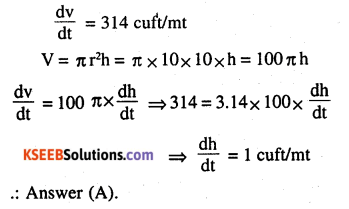Question 20.
The slope of the tangent to the curve x = t2 + 3t – 8, y = 2t2 – 2t – 5 at the point (2,-1) is
(A) $$\frac{22}{7}$$
(B) $$\frac{6}{7}$$
(C) $$\frac{7}{6}$$
(D)$$\frac{-6}{7}$$Question 21.
The line y = mx + 1 is a tangent to the curve y2 = 4x if the value of m is  ………..
(A) 1
(B) 2
(C) 3
(D) $$\frac{1}{2}$$Question 22.
The normal at the point (1,1) on the curve 2y + x2 = 3 is ……………….
(A) x + y = 0
(B) x – y = 0
(C) x + y = 1
(D) x – y = 1Question 23.
The normal to the curve x2 = 4y passing (1,2) is
(A) x + y = 3
(B) x – y = 3
(C) x + y = 1
(D) x – y = 1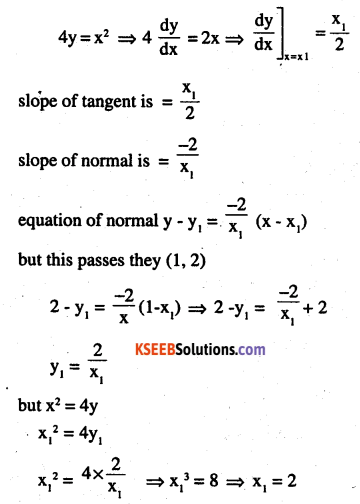Question 24.
The points on the curve 9y2 = x3, where the normal to the curve makes equal intercepts with the axes are ………………
(A) $$\left(4, \pm \frac{8}{3}\right)$$
(B) $$\left(4, \frac{-8}{3}\right)$$
(C) $$\left(4,+\frac{3}{8}\right)$$
(D) $$\left(\pm 4, \frac{8}{3}\right)$$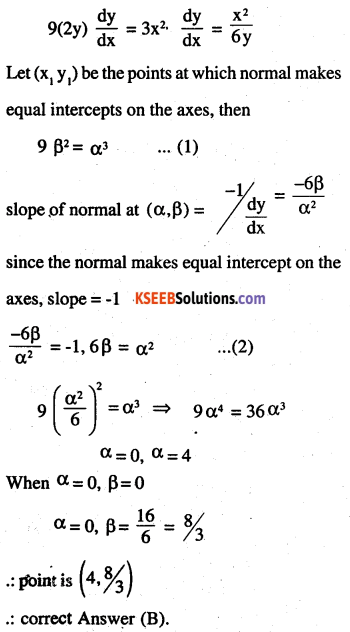### 2nd PUC Maths Application of Derivatives Miscellaneous Exercise Additional Questions and Answers

Question 1.
If the length of the three sides of a trapezium other than the base is 10cm, find the area of the trapezium when it is maximum (CBSE 2010)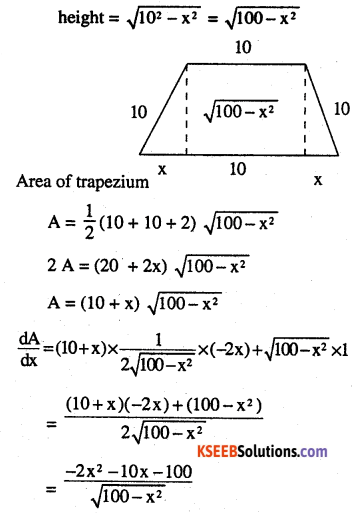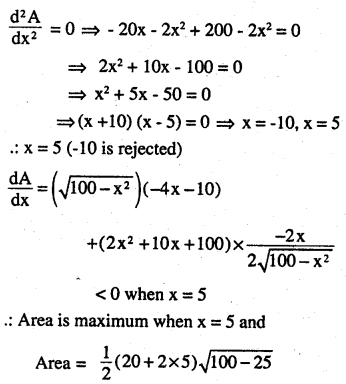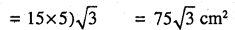Question 2.
Find the intervals in which the function sinx + cos x x ∈ [0,π] is (1) strictly increasing (2) strictly decreasing. (CBSE 2010)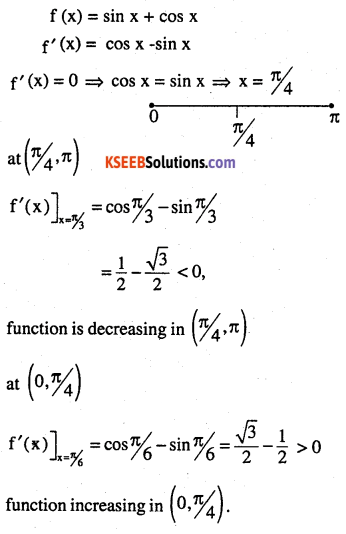Question 3.
If the radius of a sphere is measured as 9 cm with an error of 0.03 cm, find the apps error in. Calculating its surface area, (CBSE 2011)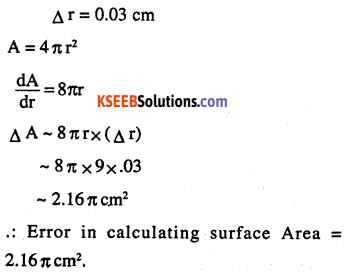Question 4.
Find the relationship between a and b so that the function of defined by
$$f(x)=\left\{\begin{array}{l}{a x+1, \text { If } x \leq 3 \text { is continous at } x=3} \\{b x+3 \text { if } x>3} \end{array}\right.$$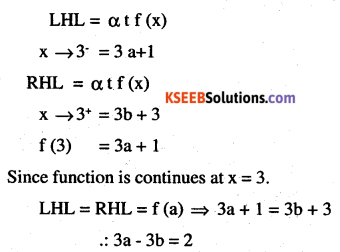Question 5.
An open box with a square box is to be made out of a given quantity of sheet of area a2. Show that the maximum volume of the box is $$a^{3} / 6 \sqrt{3}$$ (PUC 2011)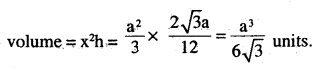Question 6.
Show that the rectangle of maximum perimeter which can be insenibed in a circle of radius a is a square of side $$\sqrt{2}$$ a. (CBSE 2008)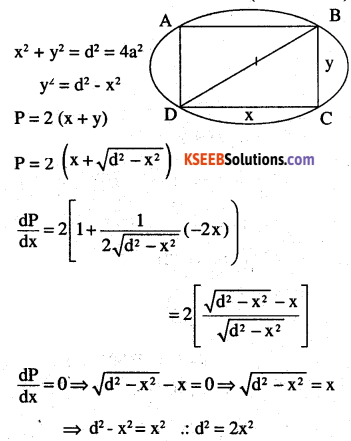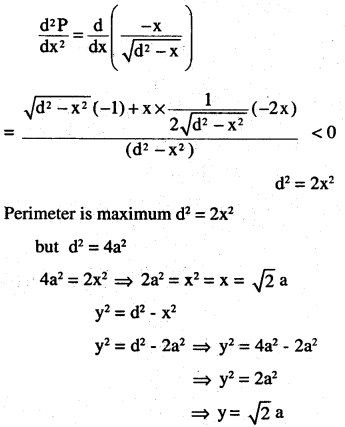$$f(x)=\left\{\begin{array}{cl}{\frac{1-\cos x}{x^{2}}} & {x \neq 0} \\{1} & {x=0} \end{array}\right.$$ (KPUC 2008)